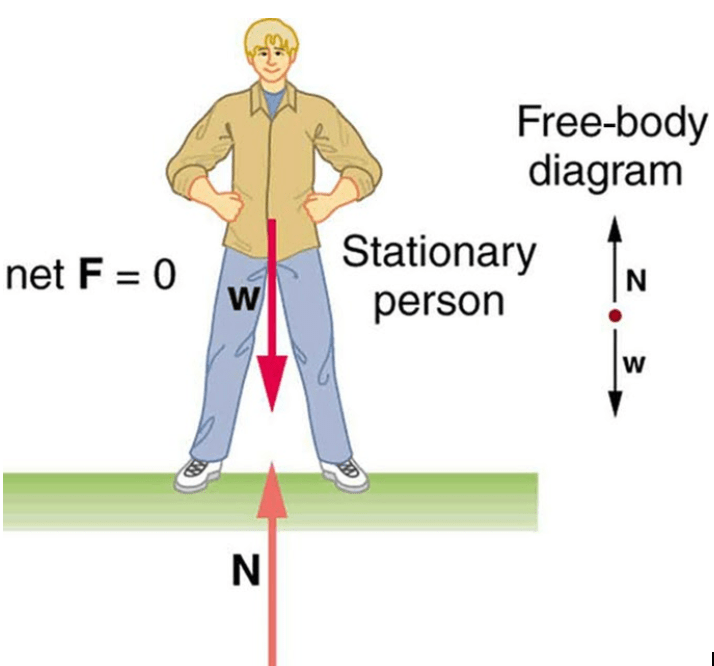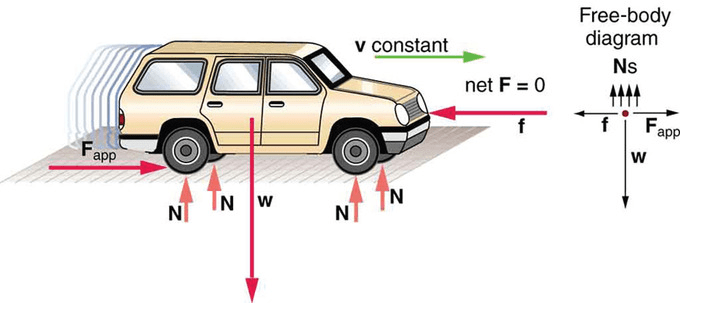Complimentary 1-hour tutoring consultation

MCAT Content / Equilibrium / Vector Analysis Of Forces Acting On A Point Object

### Vector Analysis of Forces Acting on a Point Object

Topic: Equilibrium

When an object is in equilibrium, the vector sum of all forces acting upon it is zero.

The first condition of equilibrium is that the net force in all directions must be zero. This means that both the net force and the net torque on the object must be zero. In the form of an equation, this first condition is:

Fnet = 0

In order to achieve this conditon, the forces acting along each axis of motion must sum to zero.

The condition Fnet = 0 must be true for both static equilibrium, where the object’s velocity is zero, and dynamic equilibrium, where the object is moving at a constant velocity. Below, the motionless person is in static equilibrium. The forces acting on him (both vertical) add up to zero.In another case, the car is in dynamic equilibrium because it is moving at constant velocity. There are horizontal and vertical forces, but the net external force in any direction is zero. The applied force between the tires and the road is balanced by air friction, and the weight of the car is supported by the normal forces, here shown to be equal for all four tires.Practice Questions

The mechanics of standing balance

Tension in the muscles

Patients in a wheelchair

Testing new suture material

Frictional forces on mobility walkers

An elevator in a hospital

A ramp in an administrative office

Hills, inclement weather, and cars

Coronary heart disease and blood pressure

MCAT Official Prep (AAMC)

Key Points

• There are two conditions that must be met for an object to be in equilibrium.

• The first condition is that the net force on the object must be zero for the object to be in equilibrium.

• If net force is zero, then net force along any direction is zero.

Key Terms

First condition of equilibrium: The net force in all directions must be zero.

Force: A physical quantity that denotes ability to push, pull, twist or accelerate a body which is measured in a unit dimensioned in mass × distance/time² (ML/T²): SI: newton (N); CGS: dyne (dyn)

Static equilibrium: Any system in which the sum of the forces, and torque, on each particle of the system is zero.

Dynamic equilibrium: An equilibrium(Zero Net force) with some constant/uniform speed.

Force: A physical quantity that denotes ability to push, pull, twist or accelerate a body which is measured in a unit dimensioned in mass × distance/time² (ML/T²): SI: newton (N); CGS: dyne (dyn)

Torque: A rotational or twisting effect of a force; (SI unit newton-meter or Nm; imperial unit foot-pound or ft-lb)

Translation: Motion of a body on a linear path, without deformation or rotation, i.e. such that every part of the body moves at the same speed and in the same direction; also (in physics), the linear motion of a body considered independently of its rotation.

Billing Information